# Quantitative Detection of Protein (activity)

## Experimental Background

Bovine Serum Albumin (BSA) is a protein that circulates in the blood of cows. Purified BSA can be used with Biuret solution in serial dilutions to generate a Standard Curve. The standard curve will illustrate the relationship between concentration (the dependent variable) and absorbance at 540 nm (the independent variable).  We can then use this curve to estimate the concentration of unknown samples.

1. On a graph, do you remember which axis is the dependent and which is the independent variable?
2. In the table below, can you identify which samples are the negative controls and which are the positive controls?
3. What is the prediction of the absorbance or color intensity of the different tubes?

Tags:

# pH (Activity)

## Inquire!

• What is the mechanism of action for antacids?
• Do they all antacids have the same efficacy?

## Explore: Determine the pH of common solutions

1. Use the table below to indicate whether an item is acid or base and what you predict the pH to be.:

 Solution Acid or Base Predicted pH Actual pH Coffee Cola Distilled H2O Detergent Bleach Apple Juice Antacid Solution
2. Using the pH meter or simulation at https://phet.colorado.edu/sims/html/ph-scale/latest/ph-scale_en.html, measure the pH and validate your predictions

Tags:

# Chromatography

## ChromatographyCredit:  Theresa Knott [CC BY-SA 3.0 or GFDL]Chromatography is a collective term for a set of analytical techniques used to separate mixtures. Chroma means color and graph means to write or draw. Paper chromatography is an analytical technique used to separate mixtures of chemicals (sometimes colored pigments) using a partitioning method. The paper in this method is called the stationary phase because it does not move and serves as a substrate or surface for the separation. Analytes (substances being analyzed) are separated from each other based on a differential affinity to a solvent. The solvent dissolves and carries the analytes along the matrix of the stationary phase. Since the solvent moves through a wicking action, it is called the mobile phase.

The distance that the analyte migrates along the paper related to the total distance that the solvent or mobile phase moves is called the Retention Factor or RF.Tags: ,

# pH

## Acids and Bases

We can call any compound that adds H+ ions (a free proton) into solution an acid. Along with this, we would expect that any compound that would decrease the concentration of free H+ of a solution as a base. pH is the power of H+ of a solution. We define this power as a molar concentration of H+ in solution. This concentration invariably ends up being a relatively small number (though great in absolute numbers) and is expressed as a decimal number. Because the range of the concentrations is so great, we express these numbers as logarithmic numbers to avoid writing many 0’s after the decimal and to facilitate communicating the concentration. Since these numbers are so (relatively) small, we use the negative logarithm to describe this concentration.

Mathematically defined,The pH scale ranges so that anything below pH 7 is acidic and anything above pH 7 is alkaline. So a smaller number is more acidic. But didn’t we just state that something acidic contains more H+ ions? Remember, because we are dealing with a negative Logarithm, this means the concentration is higher.

### Logarithmic Scales

If we have a quantity that is 102, we know that translates into 100. Just as if we have a quantity of 104, we know that translates into 10000. Just as it becomes inconvenient to keep writing all those 0’s, it’s really impractical to write many many 0’s after a decimal. It’s really hard to talk about too! So we likewise will express numbers like 0.0001 as 10-4. A logarithm is the reverse function of an exponent. Therefore:

••So how do we define a solution that is pH 2? Well, we already decided that this solution is below pH 7 — making it an acid. But what does this mean in terms of H+ ion concentration?
Let’s work this out algebraically:

•Let’s bring the (-) over to the other side
•Now let’s reverse the Log → base 10
•Plug in the pH → molar concentration of [H+]

As we can now see, a solution of pH 2 is acidic because the molar concentration of [H+] is 10-2mole/L or 0.01M

### Dissociation of ions: That number is small!

It’s not a small number. Remember that a mole is 6.022 X 1023. That’s a very large number! Think about it! A solution of pH 4 is acidic, but if we plug in the formula, we realize that this is equal to 0.0001M H+ – less than pH 2 at 0.01M!

But let’s compare it to the [H+] content of H2O. Now I’m going to sound crazier! Water can be thought of as being in an equilibrium where some of the molecules are ionizing and deionizing. We can express this in 2 ways:

• H2O ⇋ H+ + OH
• 2H2O ⇋ H3O+ + OH

So at any given point, a liter of H2O at neutral pH (7) has 10-7 moles of H+ ions. Incidentally, it also has 10-7 moles of OH in solution. The second expression indicates the formation of a hydronium ion (H3O+) instead of a free proton in solution. So something that is pH 2 is a stronger acid than pH 4, right? Nope. That just indicates the amount of free protons in solution. It is more acidic but acid strength means something else. When we talk about strong acids, it means that it is more likely to donate a proton to the solution because it is more likely to ionize. Let’s look at the following:

• HA ⇋ H+(aq) + A(aq)                               Where HA is an acid dissociating in solution

If this dissociation is very high, then we say that it is a strong acid. Similarly, a compound like NaOH readily dissociates completely in solution and provides OH ions that can readily remove H+ from solution –a strong base! We speak of dissociation in terms of rates and we express this as the acid dissociation constant, Ka. This is calculated using the concentrations of [H+] (proton), [A] (conjugate base) and [HA] (non-dissociated) at equilibrium:

•Just like the orders of magnitude we have when discussing pH, the rates of dissociation are more conveniently communicated on a logarithmic scale.

•Think about it this way, if the concentration of the dissociated ions is very high, the numerator in the rate is very high → Ka is great. In other words, at equilibrium, the dissociation reaction looks more unidirectional than bi-directional as the compound is readily ionized:

• HA → H+(aq) + A(aq)

On this scale, we refer to anything with a pKa < -2 as a strong acid since it will readily dissociate in solution. This form of the dissociation constant is extremely useful in estimating the pH of buffered solutions and for finding the equilibrium pH of the acid-base reaction (between the proton and the conjugate base). We can estimate the pH by utilizing the Henderson-Hasselbalch Equation:

•## Buffered SolutionsTitration of an acid by a baseA buffer is something that resists change.  A buffered solution is one that consists of a weak acid or weak base that will control the pH of a solution. Imagine a buffer to be a reservoir of available H+ or OH- ions. If a buffered solution is pH 2, adding a basic solution to it will not cause a drastic change to the pH because the reservoir of H+ will continuously neutralize the base. Eventually, this store or reservoir of H+ will be depleted. When this happens, the pH will suddenly change. The range in which acid or base is added without a significant change in pH is called the buffered zone or the buffering capacity. When this store of H+ or buffering capacity is expended, we have reached the equivalence point that describes the point at which the base has completely neutralized the weak acid.

## pH simulation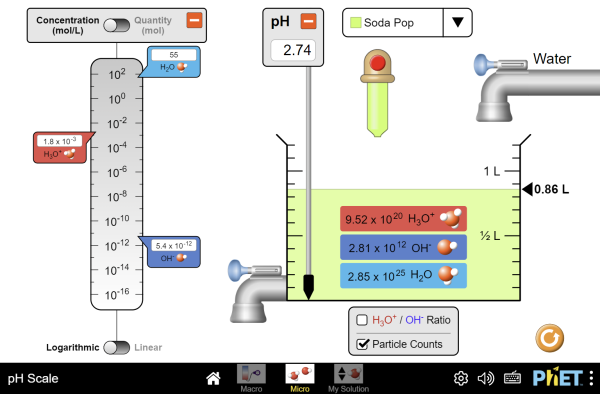Click to Run

Click on image above to run the simulation on Acids and Bases

## pH of common items

Use the table below to indicate whether an item is acid or base and what you predict the pH to be. We can determine these in class through measuring.

 Solution Acid or Base Predicted pH Actual pH Coffee Cola Distilled H2O Detergent Bleach Apple Juice Antacid Solution

Tags:

# Quantitative Skills

## Variables

Experimental science looks at cause and effect types of relationships. Controlled experiments vary one of the factors or traits  to observe the effect on another factor or trait. These factors are called variables. A dependent variable is something that is observed and expected to change as a result of modifying another factor in the experiment. That is to say, the outcome depends on another factor.  Another name for dependent variable is responding variable. The independent variable is the factor or condition that is changing or being changed by the experimenter. Sometimes waiting is the the condition that is changing, making the independent variable : time. Since we change the independent variable, it is also called the manipulated variable.

## Graphing a line

A line can be described mathematically by the equation:This is referred to as the slope-intercept form. The 2 variables y and x refer to coordinates along each axis. The term m refers to the change in the y-values over the change in the x-values. This is referred to as the slope of the line. The term b, the y-intercept, is the y-value where the line crosses the y-axis.ORThis is how the slope of a line (m) is determined.

The slope of the line indicates the relationship between the two variables, x and y. The equation of the lineindicates to us that “y” occurs as a function of changes to “x”. Sometimes this is represented by the equation. Since “y” depends on “x”, “y is the dependent variable and “x” is the independent variable.  As “x” changes, how does “y” change in response? This is what the slope reveals to us.

For more review, visit the following link.

## Slope activity

### Data Plotting Activity

1. Use the following data from the USDA to plot a and generate a trend-line.
2. Use the info from the table below or download (a text file that can be opened in notepad/textedit)
3. follow a tutorial on how to graph a scatterplot with line of best fit (trend-line)
4. or use this tutorial in plot.ly
• remember NOT to make a line graph
• Ensure that “Year” is the x-axis
• Ensure that “lbs. Mozzarella” is the y-axis
• Show the equation of the trend-line on the graph
 Year lbs. Mozzarella 2000 9.33 2001 9.70 2002 9.66 2003 9.65 2004 9.94 2005 10.19 2006 10.52 2007 11.02 2008 10.57 2009 10.63

#### What do we learn?

1. What does this scatterplot tell us about the relationship between consumption of mozzarella in relationship to years?
2. How would this graph influence the way you invest in a mozzarella cheese company? Can you predict anything about the future of cheese consumption?
3. What does the slope of this line indicate to you?
1. Use mathematics to illustrate this point.
2. The slope has a unit related to “lbs.” and “year”, what is this unit?

## Creating a Line of Best Fit

Not all points collected will fall on a straight line. A Line of Best Fit or a Trendline approximates the average of those points through a mathematical process called the least squares method. While one could “eyeball” this line, the least squares method uses the data to minimize the distance from all those points to the line to have an averaging effect.

1. Create a column of data for “x” values, “y” values, x2 and xy
2. At the bottom of these columns, sum the data. Σx, Σy, Σx2, Σxy
3. Calculate the slope from these values
•(where N=number of entries in a column)
4. Calculate the y-intercept from these values
•5. Which provides use the the functionTags: , , ,

# Quantitative Determinations

## Properties of LightCredit: Inductiveload, NASA (CC-BY-SA 3.0)Light is a type of energy that travels as a wave-particle.The wavelength of light is the distances between peaks in the waves as light travels. Wavelengths are measured in nanometers (nm) and different wavelengths of light represent differing colors. White light is a mixture of the visible light spectrum. Light of long wavelengths (infra-red) and very short wavelengths (ultra violet) are invisible to humans but can be observed by other organisms. As wavelength decreases, the energy of the light is increased.

Diffraction of light through a prism exposes the components wavelengths of light.## Spectrophotometry

Spectrophotometers (spectro-image/color ; photo-light ; meter-measure) are used for chemical analysis of solutions based on properties of absorption or transmission.Schematic of a spectrophotometer. The monochromator is a prism that splits the light. A single wave-length of light is focused through the aperture to pass through the solution in the cuvette GYassineMrabetTalk [CC-BY-SA].

Transmittance refers to the amount of light that passes through the solution.Transmittance of a light source through a cuvette. The intensity of light, I0, decreases as it passes through the solution. The light detected by the sensor, I, reflects the transmittance of the solution.

If light is being absorbed by chemicals in the solution, this results in a lower transmission. Absorbance is therefore inversely related to transmittance as expressed by the equation:Follow the virtual demonstration at: https://www.ncbionetwork.org/iet/spectrophotometer/ for a more in-depth explanation of spectrophotometry.

## Beer’s Law

Beer’s Law is a relationship between the concentration or amount of a dissolved substance in a solution that is reducing the amount of transmitted light due to the absorption of the radiant energy. Lambert’s Law states that the reduction of transmittance was related to the length of the path of light. As the light path increases through a substance, there is a reduction in transmittance. Collectively, these ideas are referred to as Beer-Lambert Law, but most observers will control the path length and simply refer to it as Beer’s Law.

Tags: ,

# Why are cells small? (activity)

## Why are cells so small?

1. Take 3 blocks of agar of different size (1cm, 2cm, 3cm) → these are our cell models
2. Measure the length, width and height of each cube using a ruler
3. Calculate the area of each face of the cubes and add all the areas together for a single cube
• a cube has 6 faces → the total surface area is the same as the area of one side multiplied by 6
1. Calculate the volume of each cube
2. Report the surface area-to-volume in the table below

Data Table: Calculating Surface Area-to-Volume Ratio

 Cell Model (cube) Length Width Height Total Surface Area Volume of cell Surface Area: Volume 1 2 3

## Stop and think:

• Which cube has the greatest surface area:volume ratio?
• Which cube has the smallest surface area:volume ratio?
• Hypothesize: In an osmosis or diffusion experiment, which cube size would have the greatest diffusion rate?

## Procedures:

1. Each group will acquire three agar cubes: A 3cm cube, a 2cm cube, and a 1cm cube. CUT AS ACCURATELY AS POSSIBLE . (This may be already completed for you.)
2. Place cubes into a beaker and submerge with 200 ml NaOH
3. Let the cubes soak for approximately 10 minutes.
4. Periodically, gently stir the solution, or turn the cubes over.
5. After 10 minutes, remove the NaOH solution
6. Blot the cubes with a paper towel.
7. Promptly cut each cube in half and measure the depth to which the pink color has penetrated. Sketch each block’s cross-section.
8. Record the volume that has remained white in color.
9. Do the following calculations for each cube and complete the following data table:

Data Table: Calculation of Diffusion Area-to-Volume

 Cube Size Cube volume (cm3) Vtotal Volume white (cm3) Vwhite Sketch cross-section of each Cube Volume of the diffused cube (Vtotal – Vwhite) Vdiffused Percent Diffusion (Vdiffused/Vtotal) % Diffused Surface Area: Volume (from previous table) 1cm 2cm 3cm

## Conclude:

• Which cube had the greatest percentage of diffusion?
• If you designed a large cell, would it be a large sphere or something long and flat?

Tags: , , ,

# Units of Measure

## The Metric System

The metric system is an internationally agreed upon measurement system based on decimals or powers of 10. Scientists use a refined version called the International System of Units (abbreviated SI). In biology, you will often find a need to describe measurements of length, volume, mass, time, temperature or amount of substance.

### International System of Units### Metric Units:

• length: meter (m)
• volume: liter (L)
• mass: gram (g)
• time: second (s)
• temperature: Celsius (°C)
• Kelvin (K) is a unit of thermodynamic temperature and is the SI unit. The Kelvin scale in the same as the Celsius or centigrade scale but offset by 273.16
• Biology uses Celsius predominantly because of the range in which organisms live.
• amount of substance: mole (mol)
• A mole is a number representing 6.022×1023 of something
• Just as a pair of shoes equals 2 shoes, a mole of shoes is 6.022×1023 shoes
• Just as a dozen eggs equals 12 eggs, a mole of eggs is 6.022×1023 eggs

Tags:

# Variable Number Tandem Repeats

## Polymorphisms

The difference in nucleotide sequences between humans lies between 0.1-0.4%. That means that people are greater than 99% similar. But when you look around the room at your classmates, you can see that that small difference amounts to quite a bit of variation within our species. The bulk of these differences aren’t even within the coding sequences of genes, but lie outside in regulatory regions that change the expression of those genes. Imagine if there were mutations to the coding sequences, this could be very deleterious to the well-being of the organism. We say that the coding sequences of genes that ultimately lead to proteins has a selective pressure to remain the same. The areas outside of the coding sequences have a reduced and sometimes non-existent selection pressure. These areas are allowed to mutate in sequence and even expand or contract. Areas of changes or differences are called polymorphic (many forms). If you were to read a repetitive set of sequences and count the repetition, you’d make mistakes and lose count. Likewise, DNA polymerase will make errors or stutter in areas of repetitiveness and produce polymorphic regions.

## Tandem Repeats

A type of polymorphism occurs due to these repeats expanding and contracting in non-coding regions. These regions are called variable number tandem repeats (VNTRs) or sometimes short tandem repeats (STRs). Any region or location on a chromosome is referred to as locus (loci for plural).  Scientists use polymorphic loci that are known to contain VNTRs/STRs in order to differentiate people based on their DNA. This is often used in forensic science or in maternity/paternity cases. Any variation of a locus is referred to as an allele. In standard genetics, we often think of an allele as a variation of gene that would result in a difference in a physical manifestation of that gene. In the case of STRs, these alleles are simply a difference in number of repeats. That means the length of DNA within this locus is either longer or shorter and gives rise to many different alleles. VNTRs are referred to as minisatellites while STRs are called microsatellites.

## CoDISDNA fingerprinting. Credit: Helixitta,The Photographer and Jeremy Seto (CC-BY-SA 3.0)The FBI and local law enforcement agencies have developed a database called the  Combined DNA Index System (CoDIS) that gathers data on a number of STRs. By establishing the number of repeats of a given locus, law enforcement officials can differentiate individuals based on the repeat length of these alleles. CoDIS uses a
set of 20 loci that are tested together. As you would imagine, people are bound to have the same alleles of certain loci, especially if they were related. The use of 20 different loci makes it statistically improbable that 2 different people could be confused for each other. Think about this in terms of physical traits. As you increase the number of physical traits used to describe someone, you are less likely to confuse that person with someone else based on those combinations of traits. Using the CoDIS loci increases the stringency since there are many alleles for each locus. The twentieth locus in CoDIS (called AMEL) discriminates between male and female.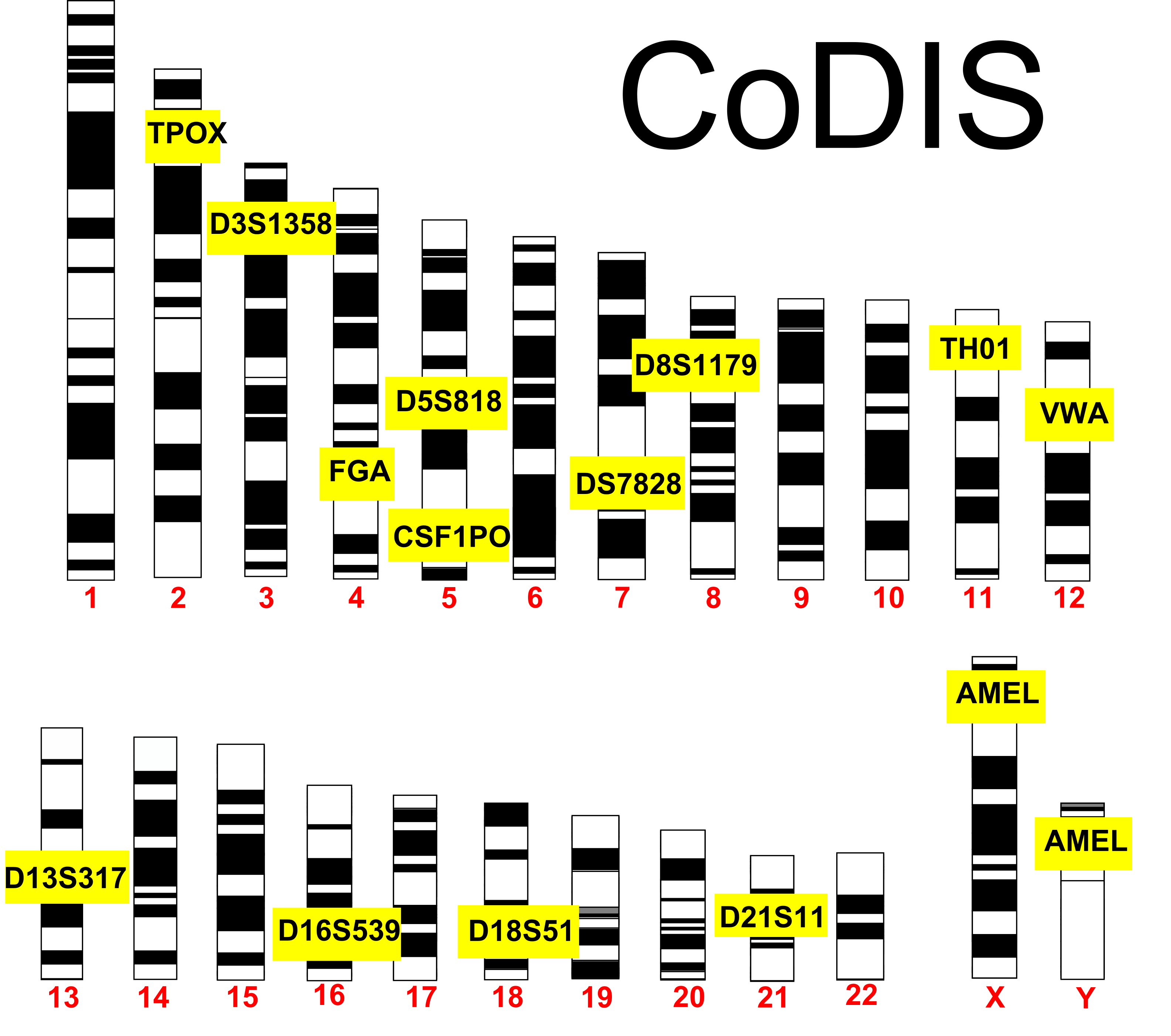CoDIS STRs: The FBI utilizes 20 different loci to discriminate between people. AMEL discriminates by gender and is located on the X & Y. Credit: Jeremy Seto (CC-BY-NC-SA)STR electropherogram of a three person mixture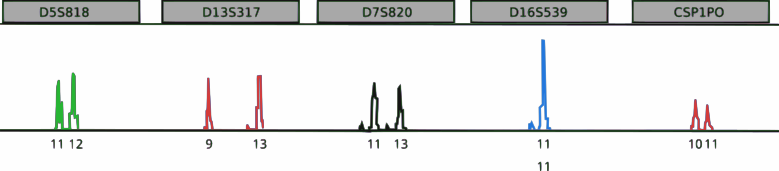A genotype of 5 STR markers. Peaks illustrate the signal detected and the intensity of the signal corresponding to repeat sizes. The peak height of D16S539 is twice the size of other peaks and illustrates that this locus contains two of the same repeat sizes.

## Crime Scene Investigation

This lab uses a CoDIS locus called TH01. TH01 is a locus on chromosome 11 that has a repeating sequence of TCAT. There are reported to be between 3-14 repeats in this locus. With the exception of X and Y in a male, all chromosomes have a homologous partner. Therefore, each individual will have 2 alleles for each CoDIS locus.TH01 STR: Outside of the STR, there is flanking areas of known sequence. The primers that amplify TH01 in PCR recognize these flanking sequences to amplify the TCAT repeats. Credit: Jeremy Seto (CC-BY-NC-SA)

At a crime scene, criminals don’t often leave massive amounts of tissue behind. Scant evidence in the form of a few cells found within bodily fluids or stray hairs can be enough to use as DNA evidence. DNA is extracted from these few cells and amplified by PCR using the specific primers that flank the STRs used in CoDIS.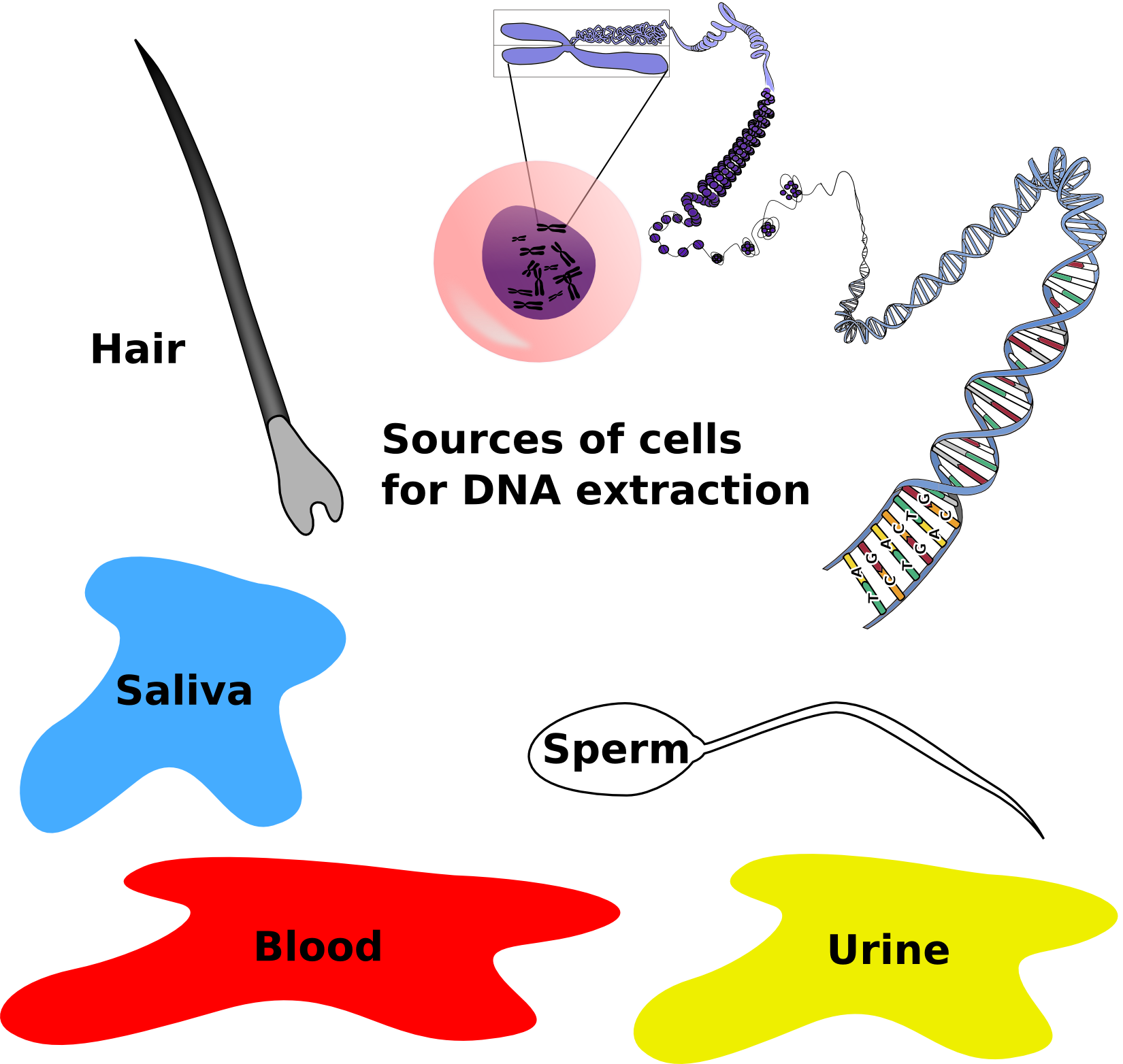DNA evidence from a crime scene: DNA can be extracted from cells found from various sources at a crime scene. PCR can amplify this small amount of DNA. Credit: Jeremy Seto (CC-BY-NC-SA)

Amplified DNA will be separated by gel electrophoresis and analyzed. Size reference standards and samples from the crime scene and the putative suspects would be analyzed together. In a paternity test, samples from the mother, the child and the suspected father would be analyzed in the same manner. A simple cheek swab will supply enough cells for
this test.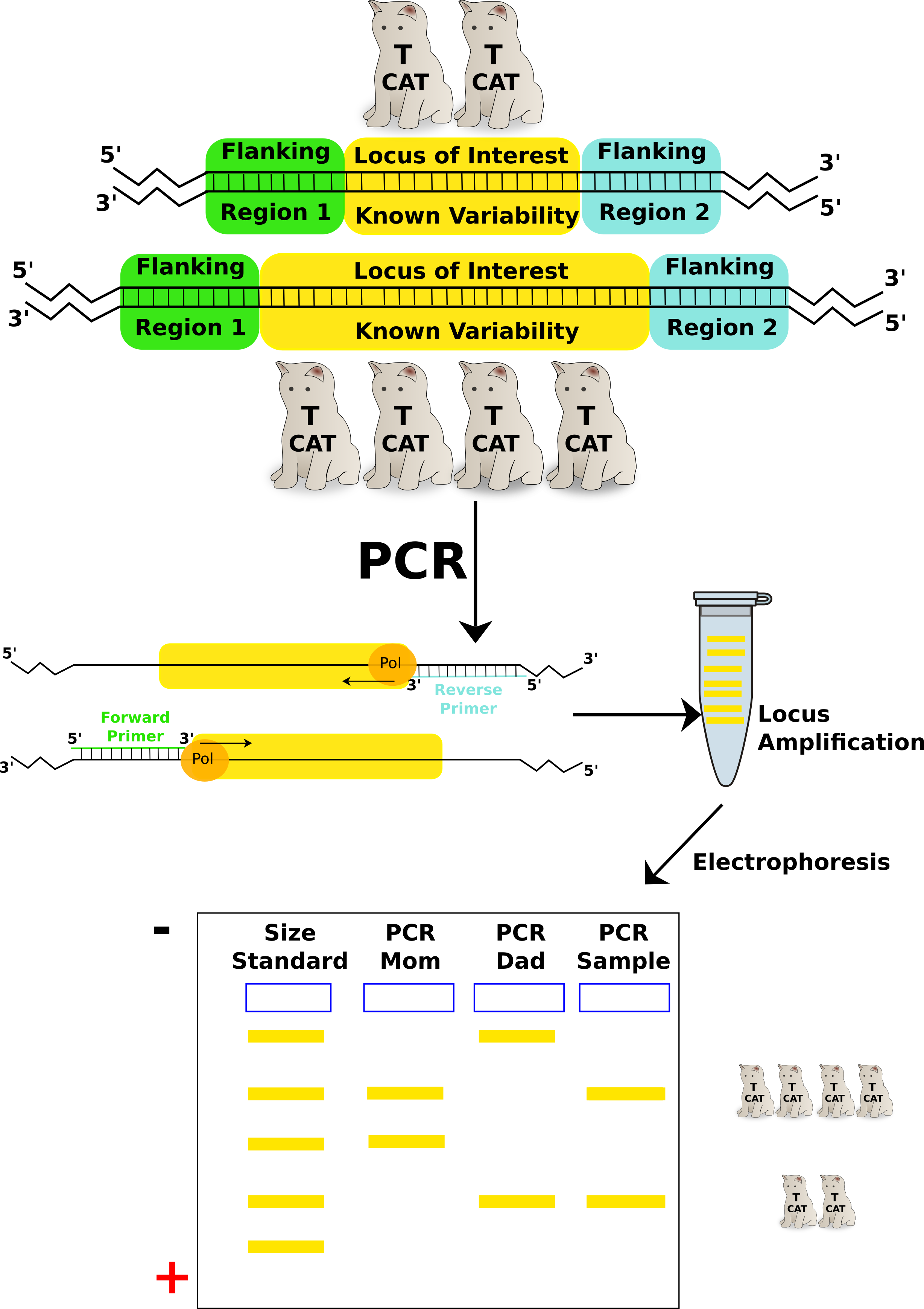TH01 locus used in a Paternity/Maternity test: Individual PCR reactions are run for each sample (mom, dad, child). The TH01 primer pair specifically amplifies the locus. Each amplified sample is run on the same gel to resolve the different alleles of TH01 from each individual. From this test the sample could be the offspring from these 2 parents but use of more STRs would make it more definitive. Count the TCATs. Credit: Jeremy Seto (CC-BY-NC-SA)

Tags: , , , ,

# Do Larger Things Diffuse Faster? (Activity)

## Activity: Do larger things diffuse faster?

Agar is a gelatinous substance derived from a structural carbohydrate found in seaweed. It is often used in cooking as a vegetarian alternative to gelatin and can be used as a thickener. Microbiologists pour plates of agar containing nutrients in order to isolate and grow bacteria and other microbes. As with gelatin, the long fibery nature of this structural carbohydrate permits it to be melted and tangled together in a mesh-like network where the spaces between molecules are filled with solution. Altering the amount of fluid solution will change the pores between fibers. More fluid will create a looser gel that has larger spaces between molecules. Reducing the fluid solution volume will conversely create a stiffer gel with smaller spaces between fibers.1. Take 2 tubes of agar and a solution of Malachite green (365 g/mole) and a solution of Potassium permanganate (164 g/mole)
2. Mark the top of the agar on the outside of the tube (the starting point)
3. Add 10 drops of malachite green to one tube and 10 drops of Potassium permanganate to the other
4. Take note of the time
5. At 20 minute intervals, measure the distance from the top that the agar has moved. Do this for at least 1 hour.
6. Plot the data and compare the trends. Describe the rate of diffusion for each.

## Stop and Think:

• Hypothesize which solution will move faster through the agar and provide a reason.

Hypothesize
which solution will move faster through the agar and provide a
reason.

Diffusion speed of dye molecules
Dye Molecular Weight Hypothesis
(fast or slow diffusion)
Malachite Green 365 g/mole

Potassium Permanganate 164 g/mole

## Conclude:

• Which solution actually moved faster?
• Did this meet your expectations?
• Propose a reason why a certain dye moved faster.
• Review through simulation

Tags: ,